#News, Views & Insights

Browse by Topic
Related Topics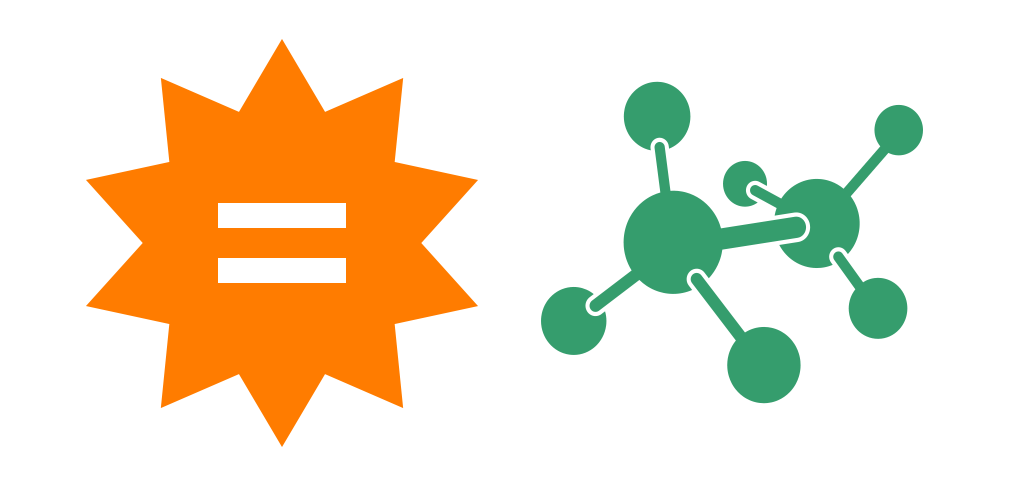Math is one of the main things that deters students from wanting to learn more about chemistry. Being a chemical engineering student, I understand this, especially for students who just have to get chemistry out of the way as a general education requirement. Essentially, step-by-step solutions are like your own on-demand math tutor: in addition to calculating the answer, Wolfram|Alpha shows you how it got there. Here are six important math skills that you will definitely use on a regular basis in your chemistry class and how they relate to different chemistry concepts.

## 1. Basic Algebra

A lot of chemistry skills involve the application of basic algebra. However, most of the algebra is hidden behind fancy chemistry words like “reactants,” “products,” “moles,” “molarity” and so on. If you read these problems closely enough, you will see that most of the work is really just an algebra problem.

First off, let’s look at an expression to brush up on algebra skills that may appear in equilibrium constant,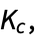problems. Simplify the following expression: (x + 1) – 2(x2 + 10 * x). This is done by distributing the negative coefficient to all parts in the parentheses, combining like terms and ordering terms from the highest exponent to the the lowest exponent. In Wolfram|Alpha, if you type “simplify (x + 1) – 2 (x^2 + 10 * x)”, you can find the step-by-step solution for this: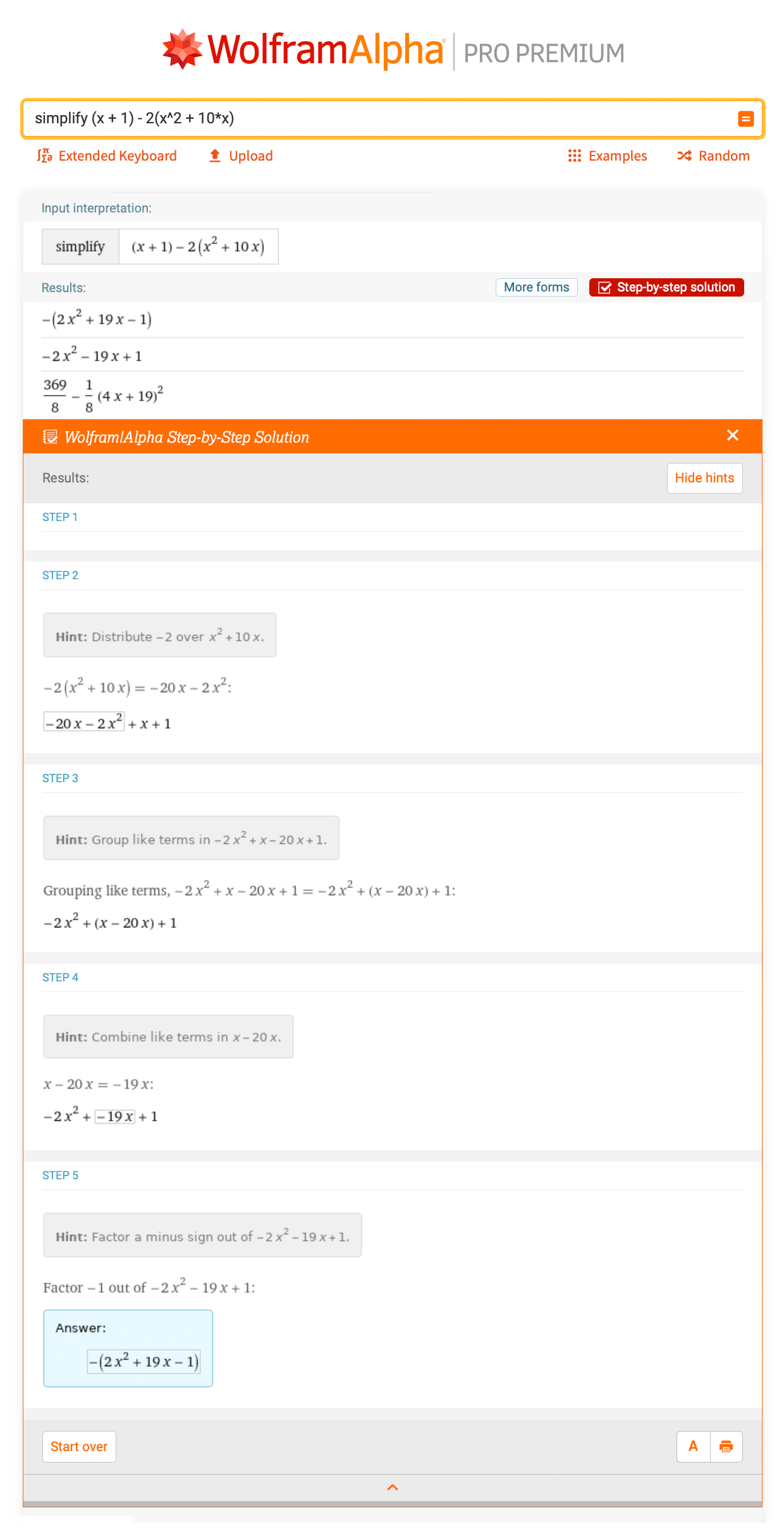Fractions are another thing that you will see a lot in chemistry, particularly in stoichiometry problems. Let’s take a look at a basic example of multiplying and dividing fractions. What is the solution for 4/3 * 2/3? Wolfram|Alpha provides a comprehensive step-by-step solution as a refresher on how to multiply fractions: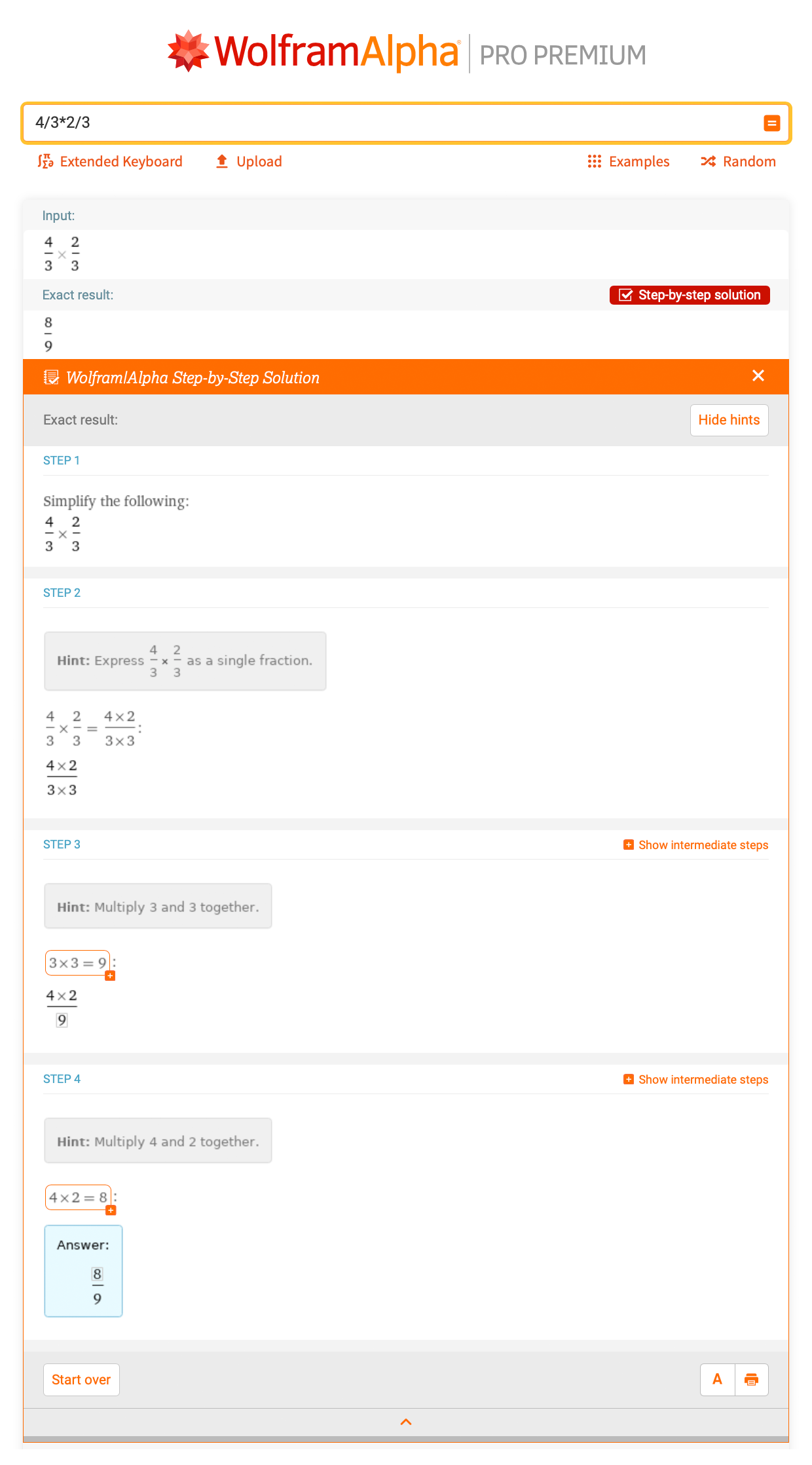Next, let’s look at a chemistry problem that uses fractions. This may be something that doesn’t look familiar to you now, but it soon will! Consider the chemical reaction AlCl3 + NaOH -> Al(OH)3 + NaCl. Assuming you have two moles of AlCl3, how many moles of NaCl can you make by running the reaction to completion? Well, you can type “2 mole AlCl3 + NaOH -> Al(OH)3 + NaCl” into Wolfram|Alpha: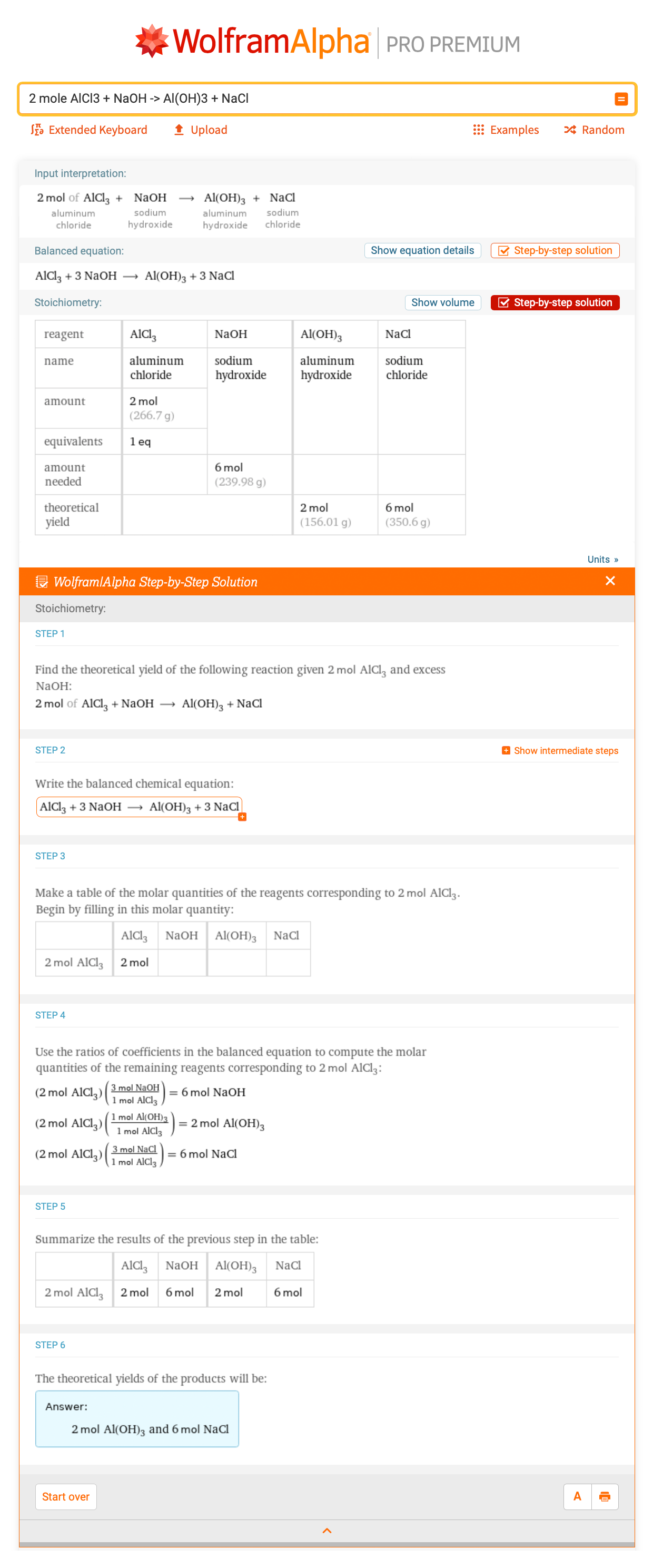Looking at the stoichiometry step-by-step results, we can see that the answer would be six moles of NaCl. Looking at step 4, your equation dwindles down to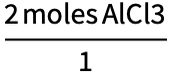*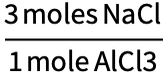= 6 moles of NaCl. Not as bad as it looks at first, right?

## 2. Unit Conversions

Every chemistry student’s worst nightmare: unit conversions, such as converting from centimeters to meters, milliliters to liters and so on. But if you think about it, they’re just fractions, which we covered in the previous section. It is one of the most common things for students to get wrong on their first chemistry exam, usually by multiplying instead of dividing or vice versa. On top of that, most units in chemistry are in metric, also known as SI, units, which may be unfamiliar to those living in the US.

Let’s start with a more familiar unit: gallons. Let’s convert one gallon of water to liters. By typing “convert 1 gallon of water to liters”, we see that it is equal to 3.785 liters. Using this knowledge, if you had two gallons, you could multiply 3.785 by 2 to get 7.571 liters: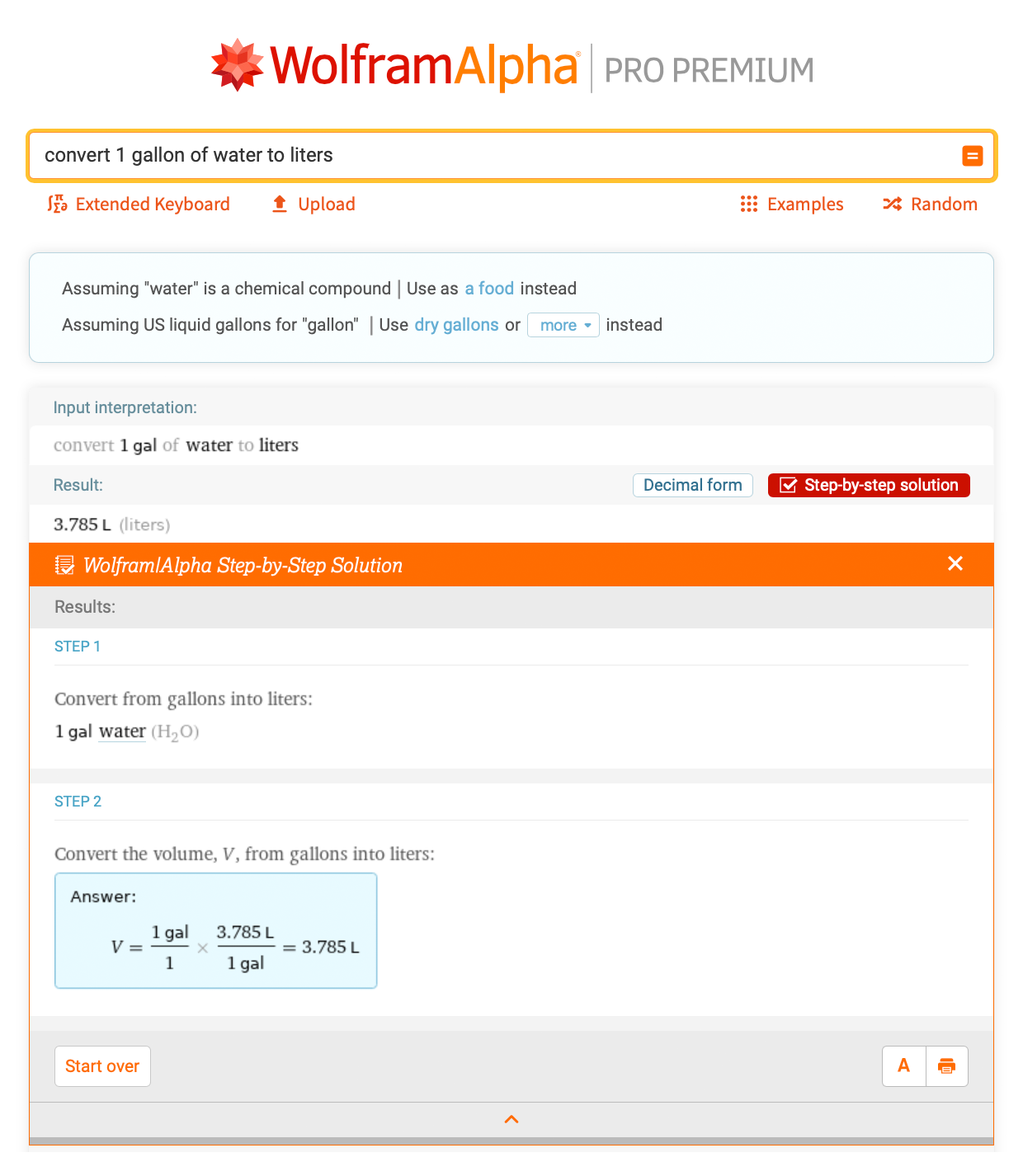Let’s look at a common chemistry conversion: converting mass to moles. This is one of many dimensional analysis problems you will encounter in chemistry. You’ve just measured out 10 g of sucrose on the laboratory balance but need to know how many moles of sucrose you have. You can do this conversion by entering “10 grams of sucrose to moles” into Wolfram|Alpha: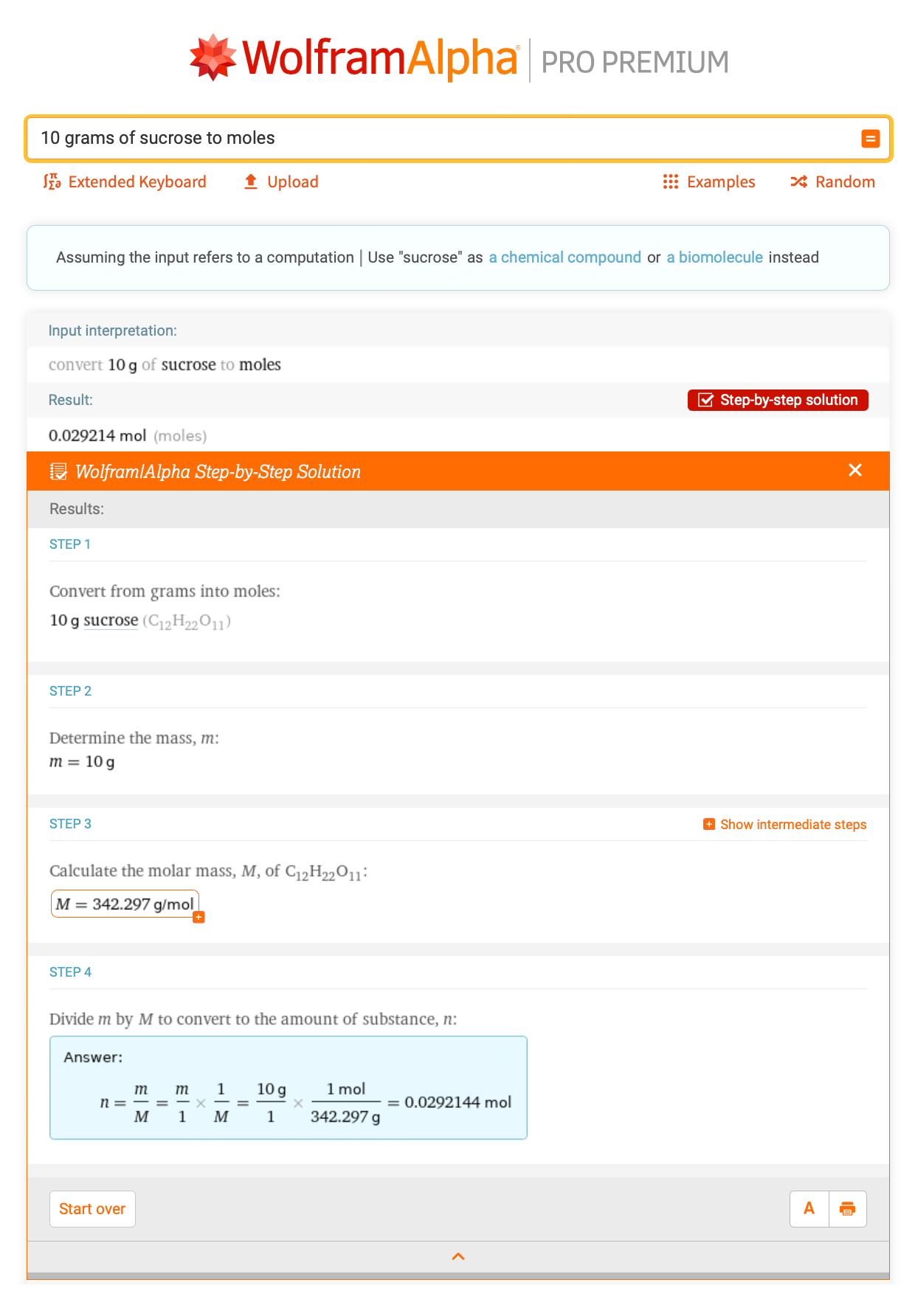## 3. Finding the Slope and Intercept of a Line

Chemists are especially fond of linear relationship, with a very popular one being the basis of Beer–Lambert law experiments. Using data collected from a spectrophotometer for samples of known concentration, a line of best fit can be formed, which is also called a linear regression. The form that this equation takes is y = mx + b, where m is the slope and b is the y intercept.

By taking a simple equation like y = 3x + 1, identifying what the slope and intercept are will be helpful as these values can be plugged into other equations or interpreted to determine specific trends or correlations. For example, if the slope is negative, that means there is an inverse correlation, versus a positive slope having a direct correlation.

Given a list of points {{1, 2}, {2, 3}, {3, 5}, {4, 6}}, Wolfram|Alpha can determine the best-fitting linear equation: “linear equation {{1, 2}, {2, 3}, {3, 5}, {4, 6}}”: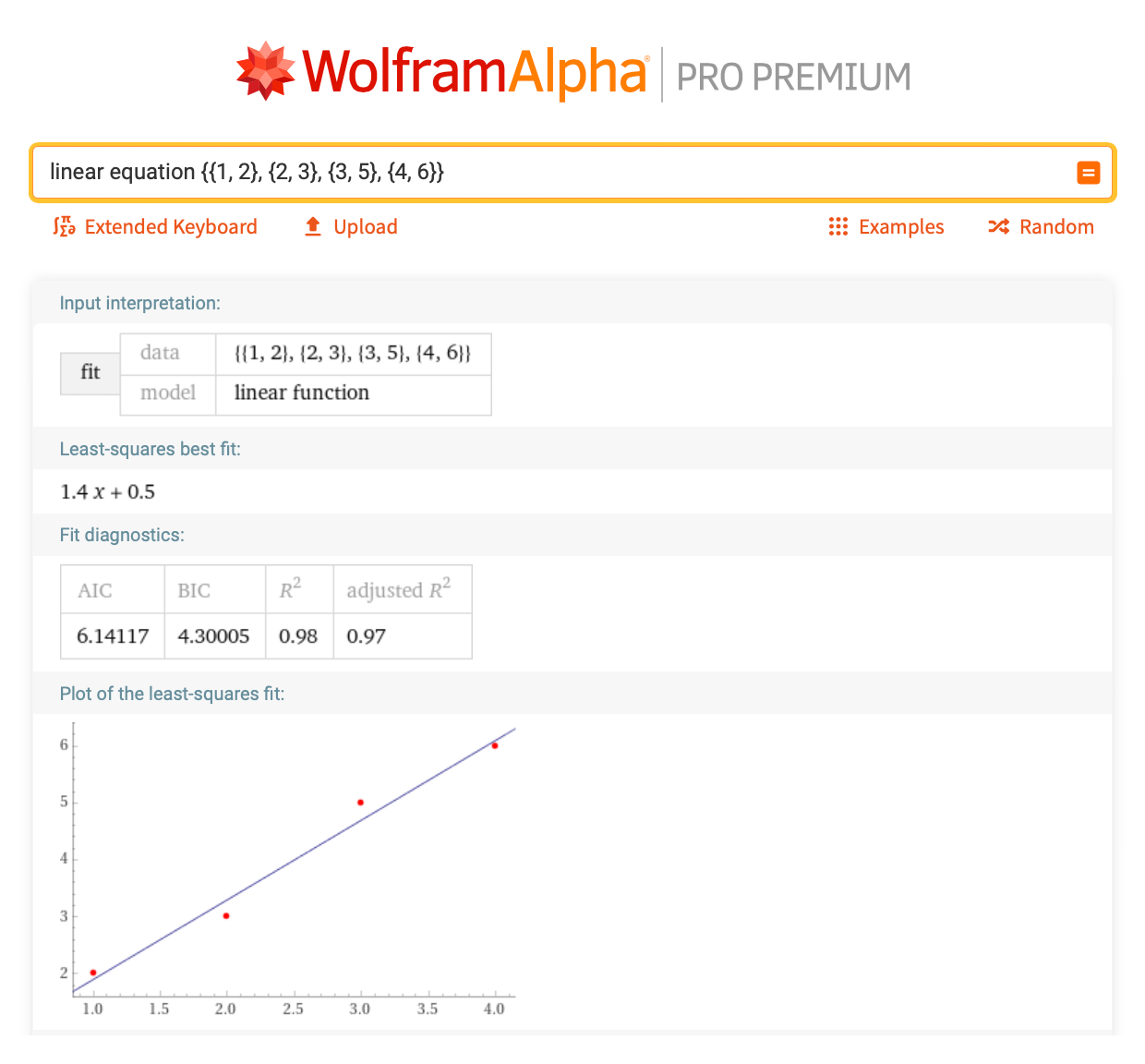Additionally, Wolfram|Alpha can be used to rewrite an equation that is not in y = mx + b form to turn it into this form. For example, given the equation 2x + 7y – 5 = 0, what is the slope and intercept? Just enter “solve 2x + 7y – 5 = 0 for y”: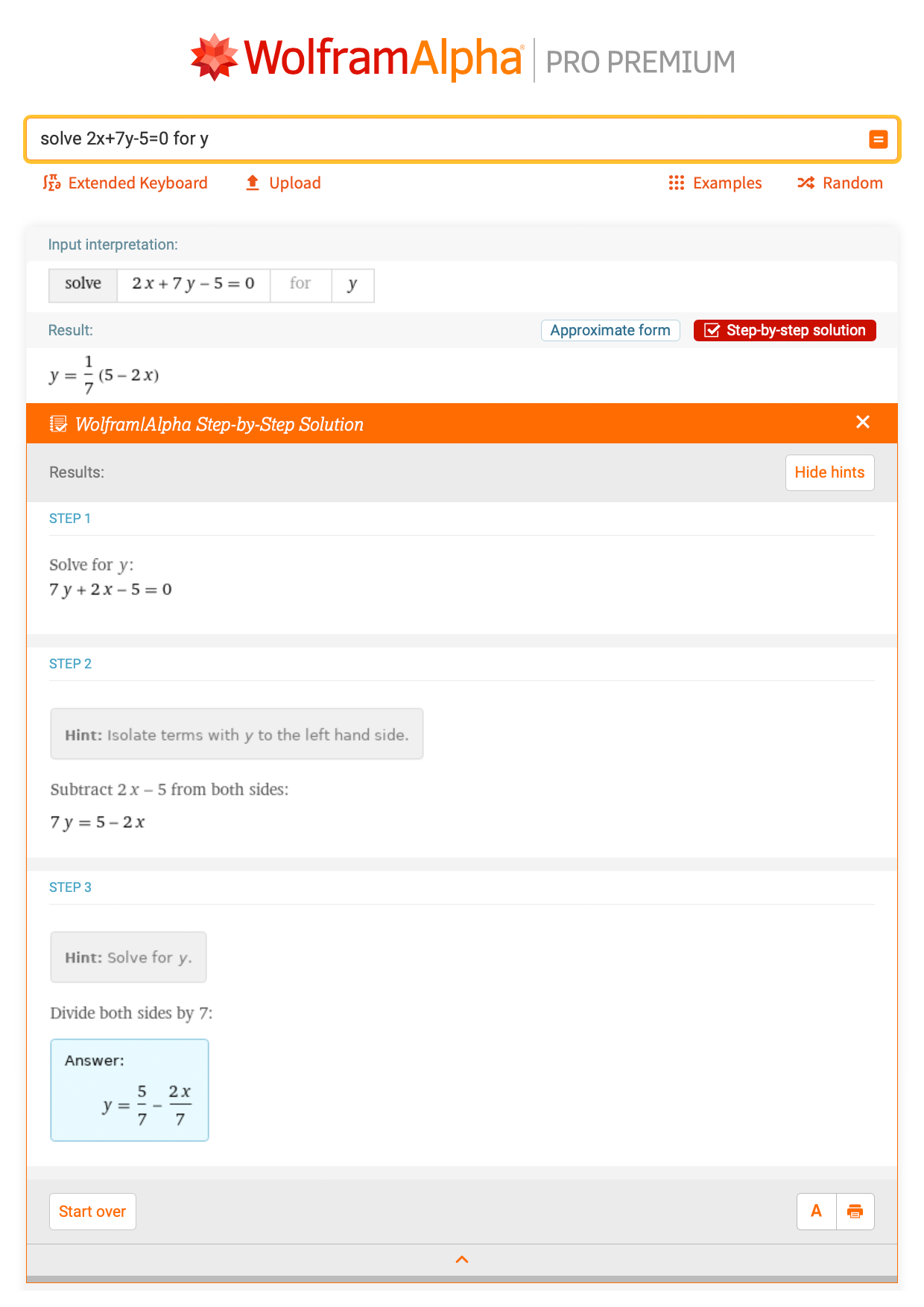The slope would be m = –2/7, and the y intercept is b = 5/7. You can also type in “slope and y intercept of 2x + 7y – 5 = 0” and receive the answers directly, but it’s helpful to know how to identify each part in the slope-intercept form of the equation. Following this, if someone were to ask what the value of y is given that x = 5, by evaluating –2/7x + 5/7 at x = 5, the answer would be –5/7, which can be calculated using the basic algebra skills seen previously: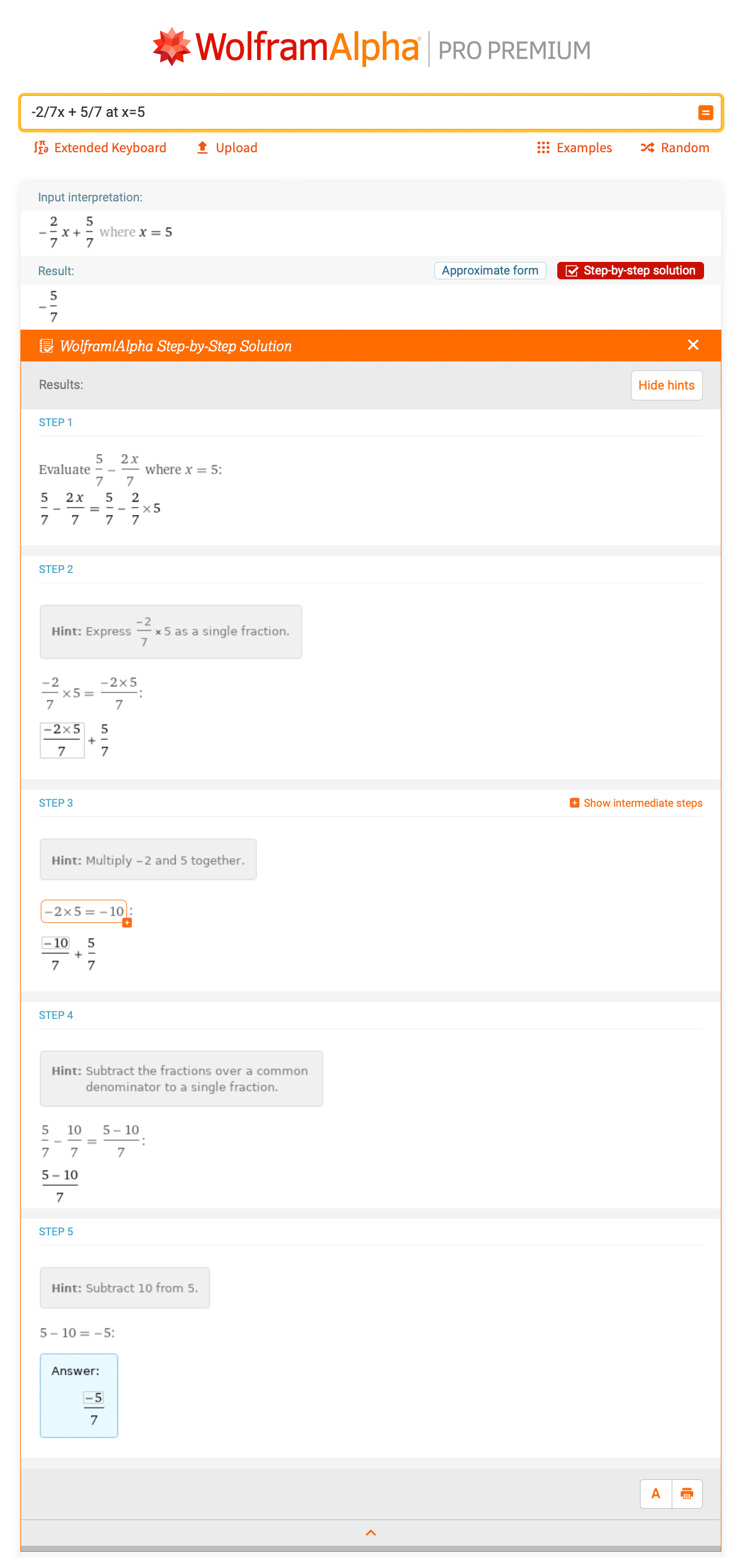Being able to identify what the slope and y intercept are, and also plugging in values to determine what y is, is a very important skill for topics in chemistry, such as kinetics.

In chemistry, we use Celsius to measure temperature instead of Fahrenheit. Given a couple known temperatures in Celsius and Fahrenheit, how can we convert other temperatures without using a digital assistant? Well, let’s take five points, where the pairings will be listed as {Fahrenheit, Celsius}: {{40, 4.4}, {50, 10}, {60, 15}, {70, 21}, {80, 26}}. We can find the information for this line with “linear regression {{40, 4.4}, {50, 10}, {60, 15}, {70, 21}, {80, 26}}”: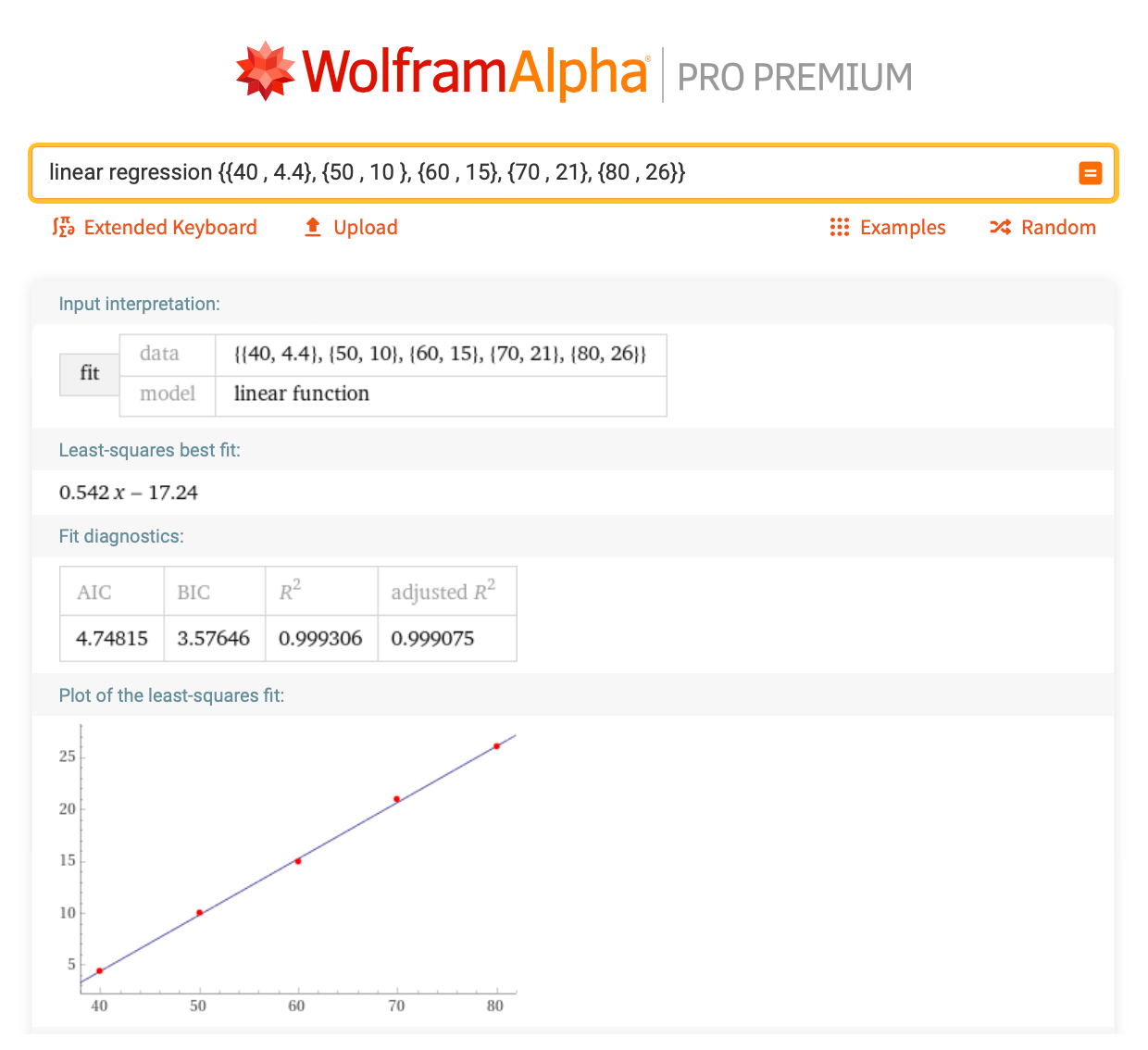The equation of this line is y = 0.542x – 17.24, with x being Fahrenheit and y being Celsius. So if you have a value in Celsius or Fahrenheit, you can convert to either one by plugging the value into this equation and solving for the other variable. So if you’re trying to convert from Fahrenheit to Celsius, of course just typing in “75 F to C” will do the trick, but being able to see this linear correlation can help you determine this conversion even if you don’t have access to the internet.

## 4. Solving for x

Another important concept needed to succeed in your first chemistry class is how to solve for x using inverse operations. Rearranging numbers and variables around to isolate x and determining the unknown value are so important in solving for different values, such as energy, mass, volume and so on.

Let’s take an example using multiplication and division to solve for x. Given the equation 8 * x = 3 * 0.08206 * 298, what is the value of x? This is actually an equation taken straight from the ideal gas law with numeric values plugged in. Ideal gas law problems allow us to solve for pressure, volume, amount of substance and temperature. In all cases, the effort distills down to an expression with x in it to be solved for. After combining terms, Wolfram|Alpha solves this via “solve 8 * x = 3 * 0.08206 * 298 for x”: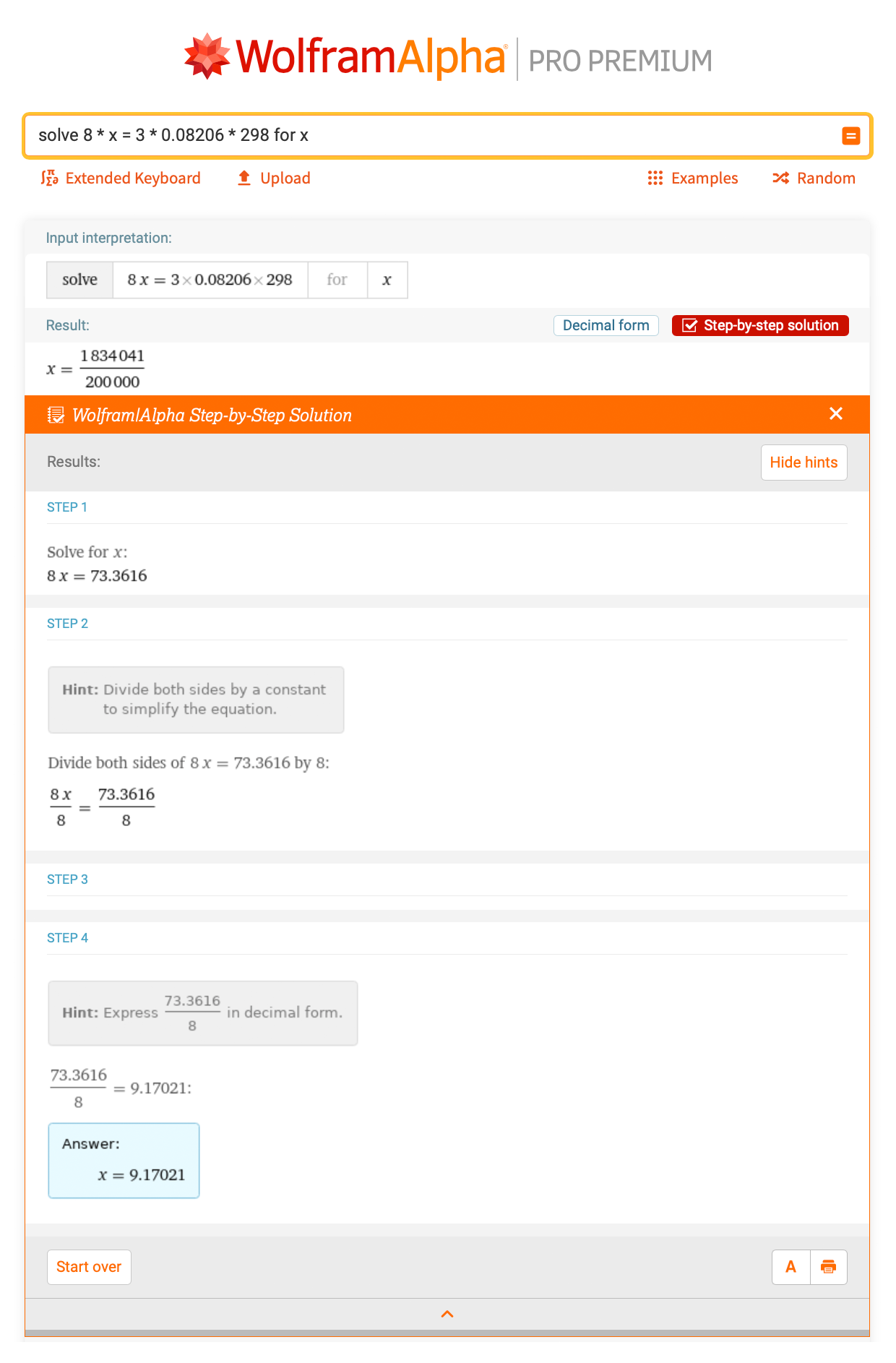We could include results from the ideal gas law calculator for an example problem. Determine the volume of 1 mole of methane gas at 150 Kelvin and 1 atm: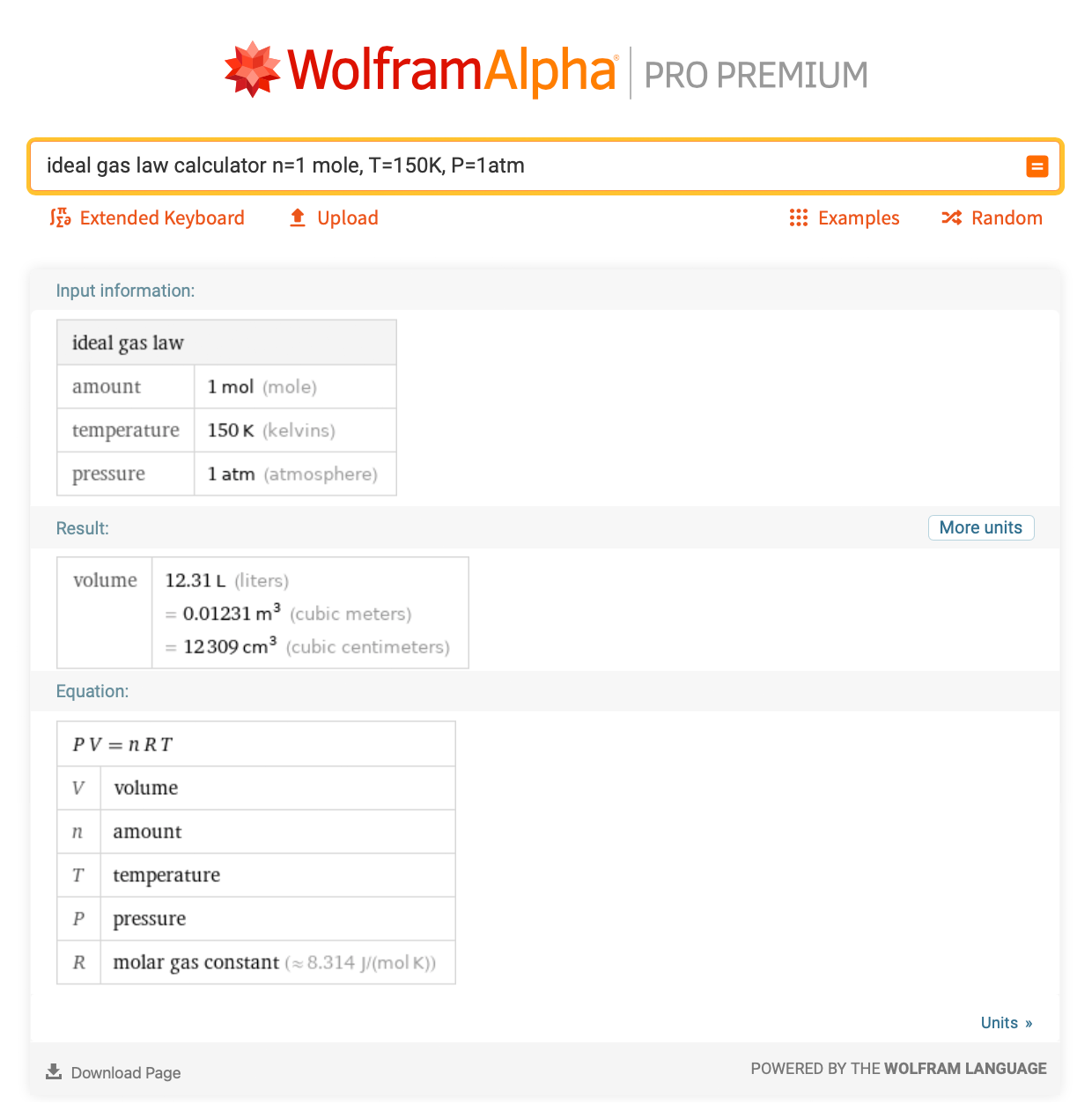Here is another problem that uses exponent rules: “solve for x when 10^2 = 200/sqrt(x)”. After simplifying 102 to 100, the rest of the steps are shown, which gives a good refresher for using exponents to isolate variables: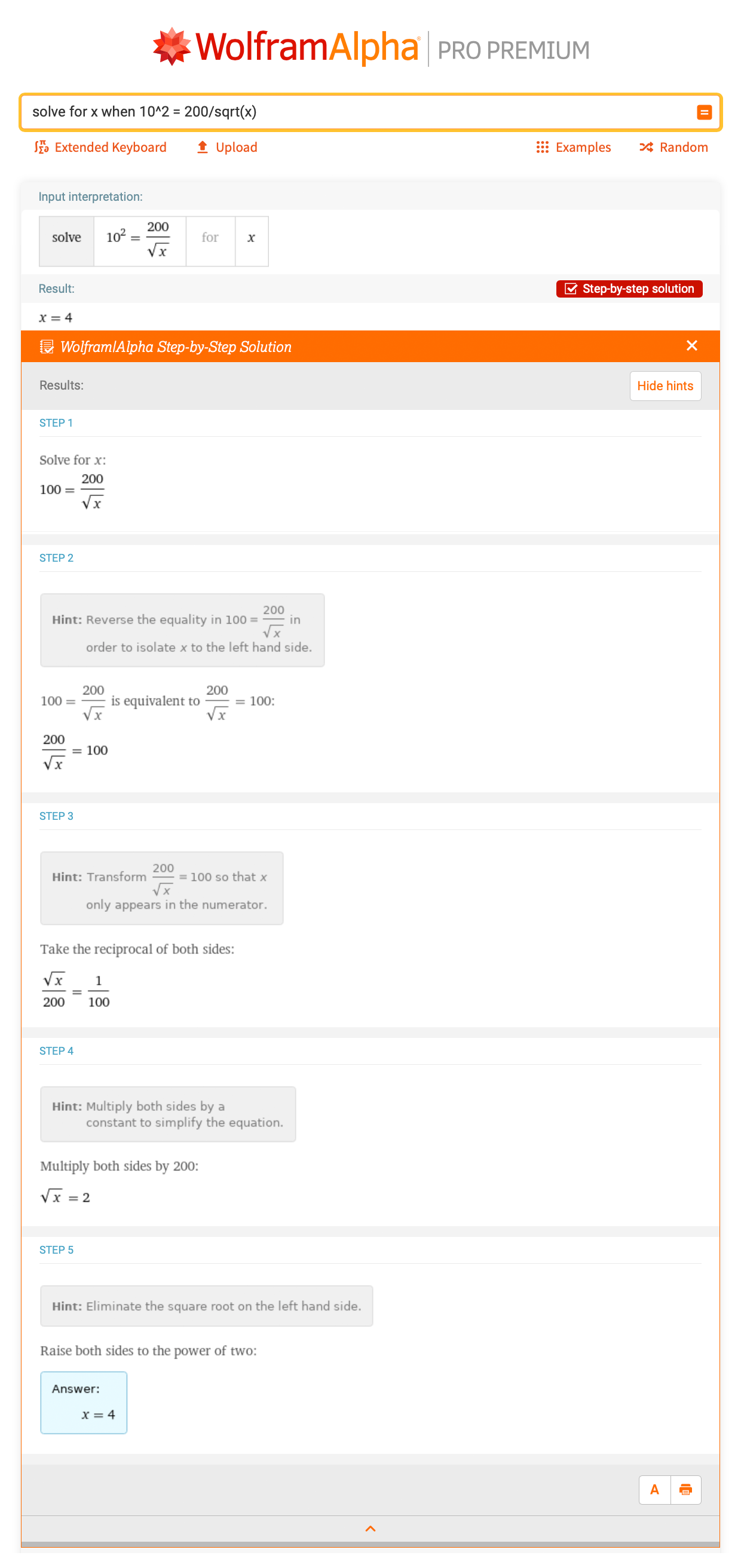Let’s take a look at a more complicated example. This one involves solving for x in a quadratic function, which appears in equilibrium and buffer problems. Given that 0.0125 = (x)*(x + 0.1) / (3 – x), what is x? This one is much more difficult to do by hand, so let’s look at what Wolfram|Alpha has to say for “solve 0.0125 = (x)*(x + 0.1) / (3 – x)”: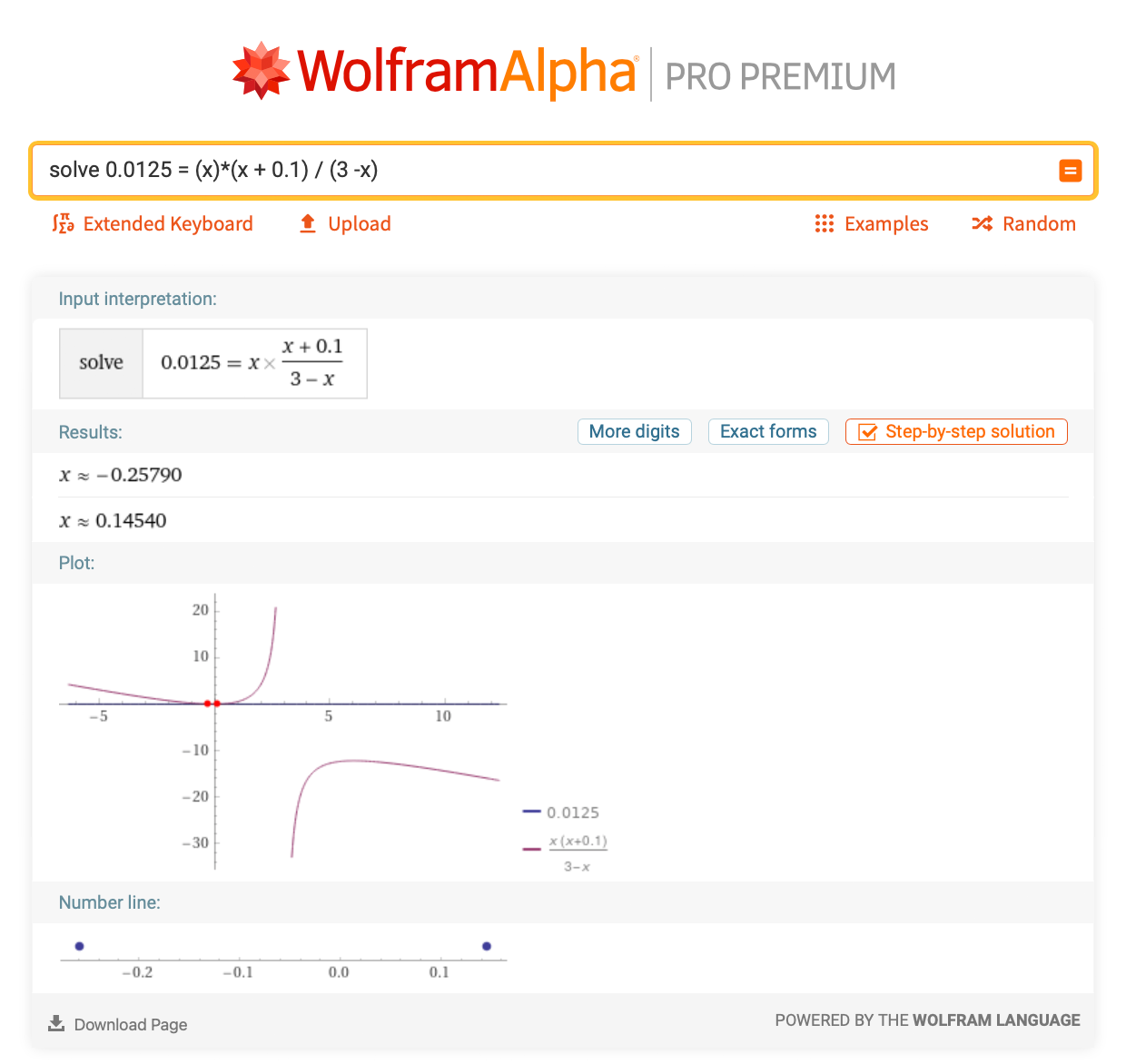There is a step-by-step solution available for this problem as well, but the two answers after clicking the Approximate form button are x = –0.25790 and x = 0.14540. This is very useful in chemistry, especially when solving equilibrium reactions. However, you will encounter solving for x anywhere in chemistry that involves an equation.

## 5. Logarithms

For the most part, logarithms are computed using calculators when solving acid-base problems, but it is helpful to know how to use them. In chemistry, the main types of logarithms that are used are base 10 and base e, or the natural log (the ln button on your calculator). Unless the logarithm is equal to a whole number, you would probably have to use a calculator to solve these. An important concept to know about logarithms is how to switch back and forth from the exponential form to the logarithmic form. For example, what is the exponential form of log10(100) = 2? It would be 102 = 100. Given this example, you should be able to determine the value of a variable given a logarithm problem. For example, what is the value of x in log10(x) = 4? Wolfram|Alpha has a great step-by-step solution for this: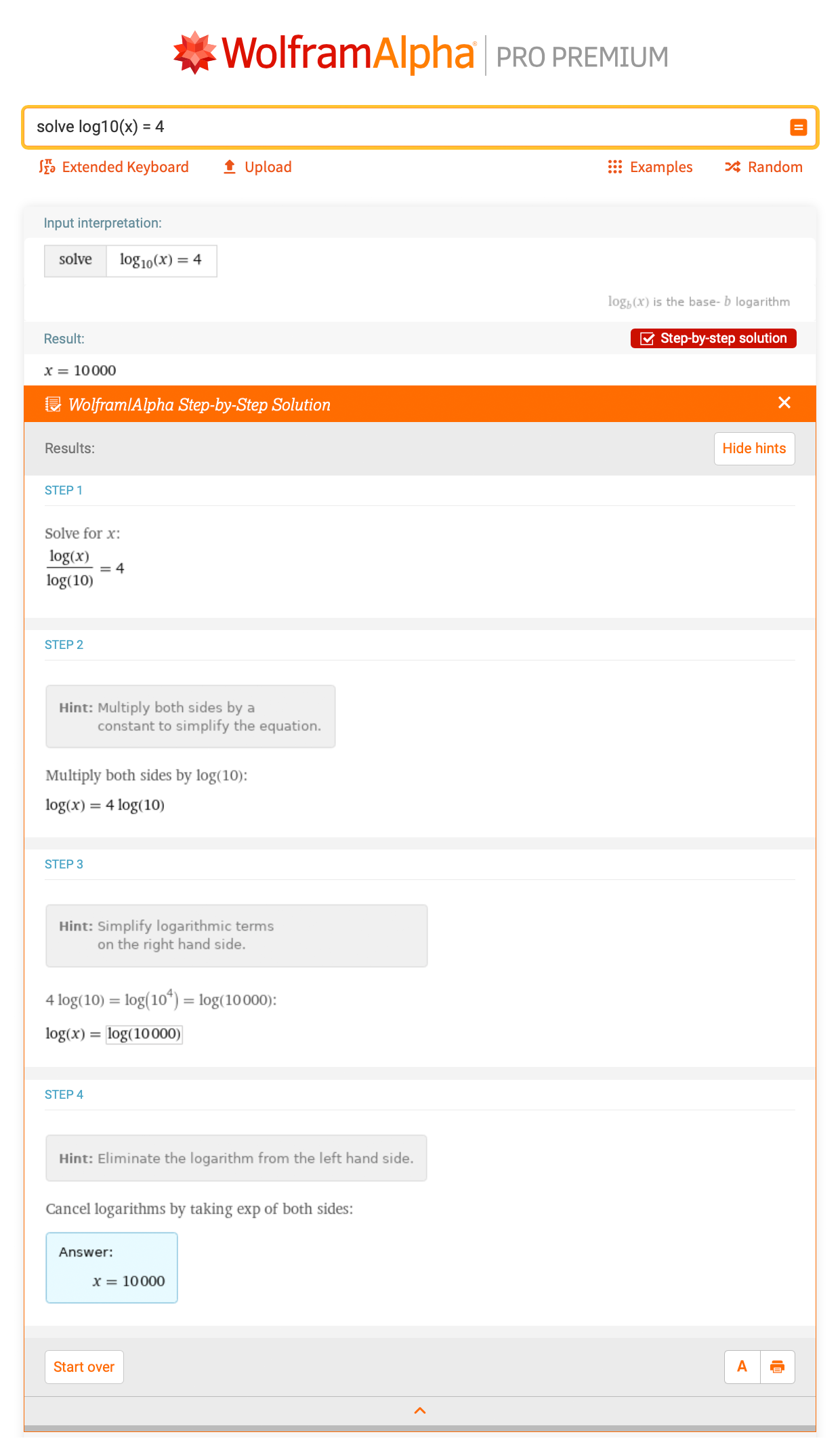Logarithms are mainly used in pH calculations, since pH is measured on a base-10 scale, meaning that a pH of 3 is 10 times “stronger” than a pH of 4. That being said, many times you would end up with a pH that is not a whole number. You can also use Wolfram|Alpha to calculate logarithms that would be harder to compute by hand, such as the value of x in the equation log10(x) = 3.5. “Solve log10(x) = 3.5” generates an answer of 3162.3, as seen here after clicking the Decimal form button: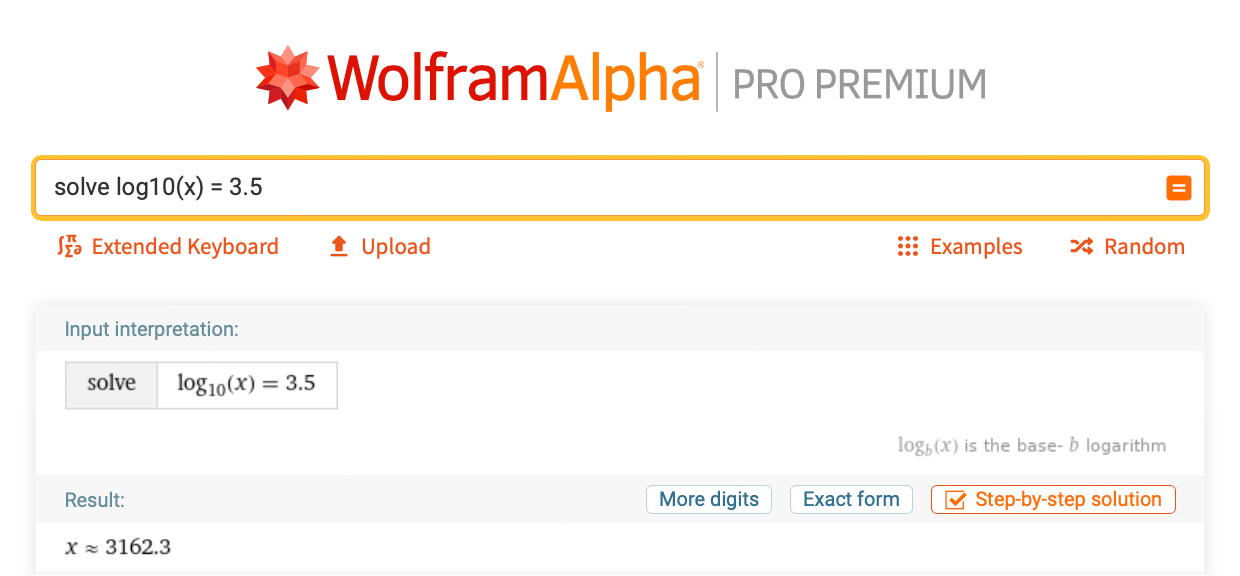The process for solving this problem is similar to the previous one, but it is very hard to calculate a decimal exponent, so using a computer or calculator to determine this answer is necessary. It also is worth mentioning that log(x) in Wolfram|Alpha is not interpreted as base 10, but as the natural log. If you need base 10, make sure to type log10(x).

## 6. Finding the Roots of a Quadratic Equation

The last important concept to know for your first chemistry course is finding roots of quadratic equations. A quadratic equation has the form a * x2 + b * x + c = 0 with the values of x given by –b ± Sqrt[b2 – 4ac]/2a, which is known as the quadratic formula. Of course, solving for roots like this often trips people up, but using the computer to solve it for you is much quicker and exact! In the world of chemistry, solving for the roots of a quadratic function is useful when solving equilibrium problems, particularly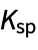equations, where there is no denominator like there usually is.

Consider theproblem where you need to solve for the roots for the equation x * (0.001 + x) = 1.5 * 10–5. This would be a case where the terms have to be rearranged first so that the equation is equal to 0 before using the quadratic formula. There is a detailed step-by-step solution for this problem: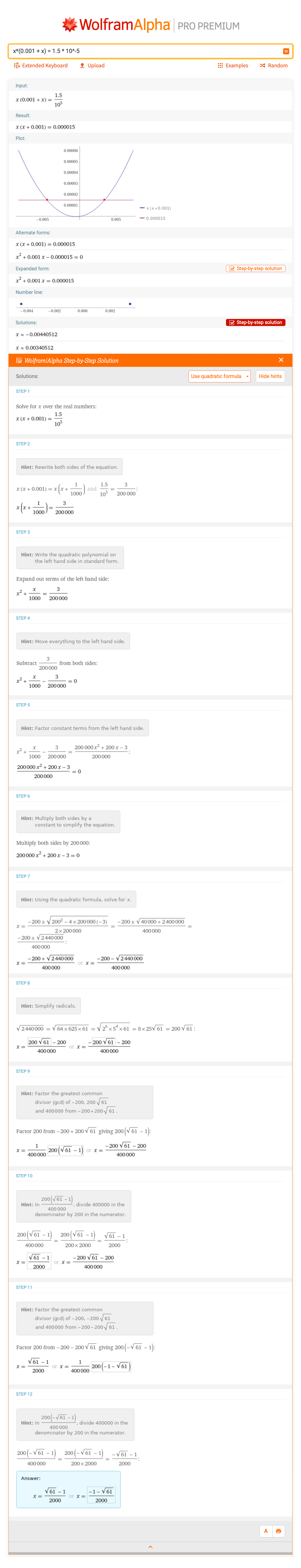Sometimes equations containing cubic terms, like x3, and higher may appear in your homework problems. An example of this is x3x2 + 9 * x – 9 = 0. What are the values of x in this case? Wolfram|Alpha will kindly determine the roots with the input “solve x^3 – x^2 + 9 * x – 9 = 0”: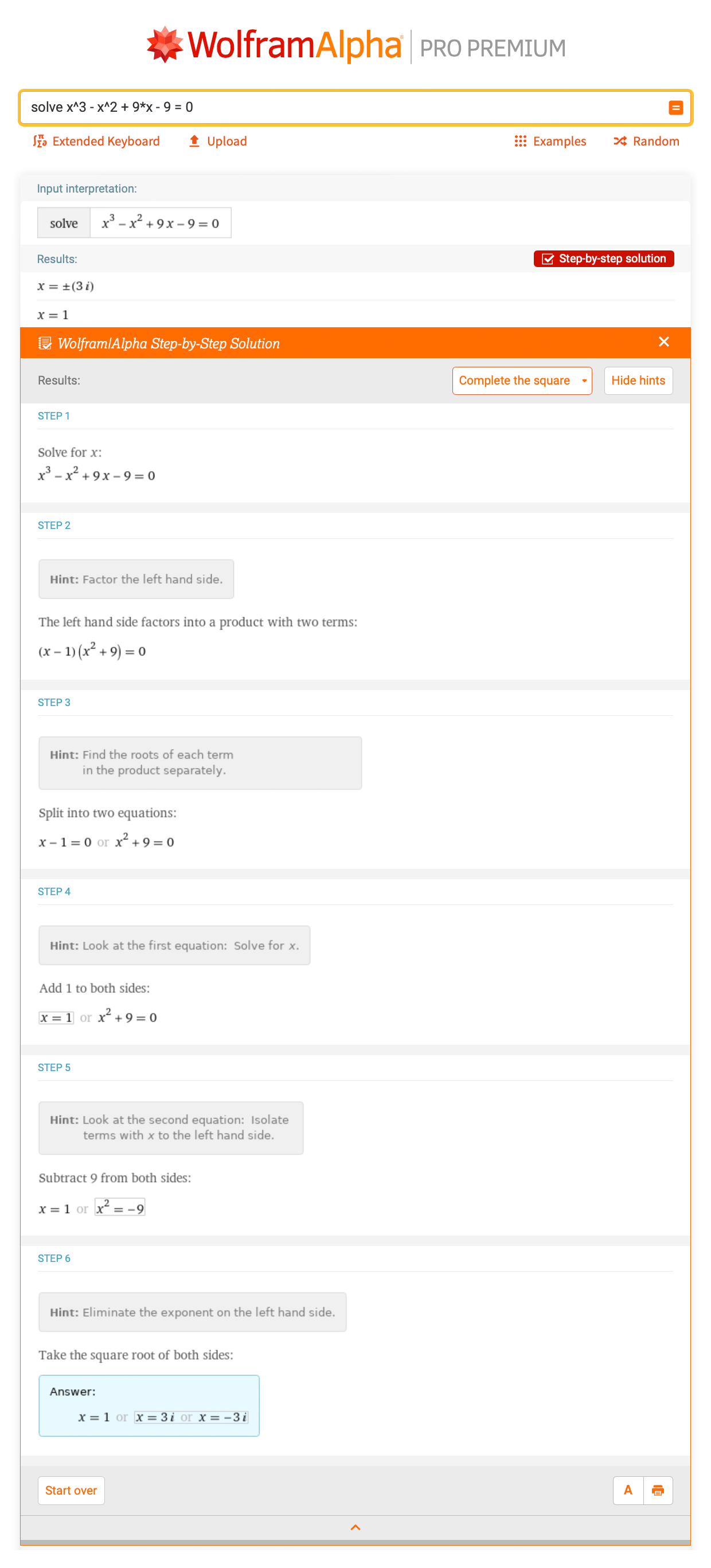Keep in mind that there are more ways to solve for the roots of an equation than the quadratic formula. Wolfram|Alpha provides different types of step-by-step solutions that can teach you to solve the same equations by hand, whether it be factor method, quadratic formula or completing the square.

## Math and Chemistry Go Hand in Hand

As you learn more about chemistry concepts, you can see how intertwined the math is with chemistry. It really is beautiful how it all comes together in the end, but it’s important to be comfortable with the algebra behind it in order to be able to see the connectedness. I recommend using Wolfram|Alpha Pro for solving both math and chemistry problems, since many of the problems will give you the step-by-step solutions for solving them, which can help you have much success in your chemistry classes!

For more chemistry-focused step-by-step solutions, see these posts from the Wolfram|Alpha Chemistry Team: